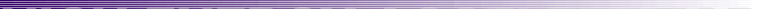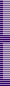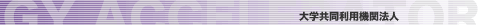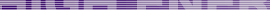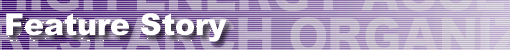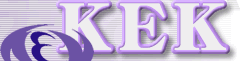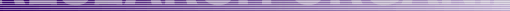>Top >Feature Story >this page last update: 10/05/19Superstring theory: stringing together all the forces of physics
KEK scientists developed the IKKT matrix model
that is used to make predictions from the Type IIB
contributions of KEK’s superstring theorists in
the field, and how superstring theory, the possible
Theory of Everything, is starting to make
predictions that can be tested by experiment.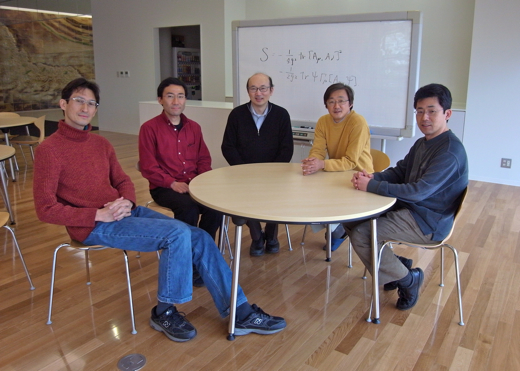Five theorists at KEK are at the leading edge of superstring theory. From left: Dr. Shun’ya Mizoguchi, Prof. Jun Nishimura, the leader of the superstring group Prof. Yoshihisa Kitazawa, Prof. Satoshi Iso, and Dr. Makoto Natsuume.

“The answer to the Ultimate Question of Life, the Universe, and Everything is” probably not forty-two. The supercomputer Deep Thought created by hyperintelligent pandimensional philosophers in Douglas Adams’ the Hitchhiker’s Guide to the Galaxy didn’t know what the question was anyway. Luckily, physicists on the planet Earth may have known more sensible questions to ask, such as, Is there an ultimate theory that governs our universe, and if so, what is it?

For the past half-century, physicists have tried to find a theory that unites the two central theories of modern physics: quantum field theory and Einstein’s theory of general relativity. This hypothetical theory is popularly referred to as the theory of quantum gravity. The two current central theories have been vastly successful, surviving many experimental and observational tests across vast distance scales. However, these two theories cannot be easily reconciled as their fundamental notions of spacetime differ. At microscopic scales, the uncertainty principle of quantum field theory states that every point in space is subject to riotous quantum fluctuations, in which elementary particles and antiparticles are continuously created and destroyed. This seething sea of vacuum cannot be reconciled with the smooth curves of spacetime described by general relativity.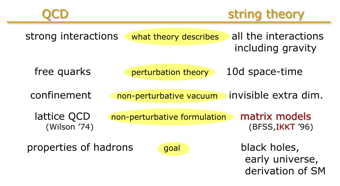String theory was originally formulated to describe the strong interaction. However, the theory of quantum chromodynamics (QCD) soon took its place. Although temporarily abandoned, string theory later became the most promising candidate for a theory of quantum gravity.On the one hand, general relativity explains gravity by means of curvature of spacetime. On the other hand, quantum field theory explains the other three forces—strong, electromagnetic, and weak—as the result of interactions among fundamental particles using something called gauge theory. “We need a theory that can explain both gravity and gauge interactions,” says Prof. Yoshihisa Kitazawa of KEK, the leader of the superstring group at the KEK Theory Center. “One promising candidate is superstring theory.”

String theory proposes that the elementary constituents of matter are one-dimensional strings, rather than zero-dimensional particles. These strings may be either open or closed, and may vibrate at different frequencies. The shape of the string and the frequency of the vibration determine the type of the particle. “Consider Newton’s law of gravitation. As you move closer and closer to a point mass, the gravitational force between you and the mass becomes infinitely large,” explains Kitazawa. This creates a problem because a singularity—divergence to infinity at zero radius—is a discontinuity in spacetime. “The extension of particles to strings is the simplest way to avoid the singularities in spacetime that are inherent in the point particle picture.”

String theory was originally developed to explain strong interactions, but was replaced by the successful theory of quantum chromodynamics (QCD). It was not until 1969 that Yoichiro Nambu and others independently formulated string theory as quantum mechanics of one dimensionally extended object. In the mid 70s, Japanese physicist Tamiaki Yoneya found that string theory predicted the existence of a graviton—a hypothetical elementary particle that mediates gravity. This was the beginning of a unified field theory, a single theory that could describe the functioning of all four fundamental forces.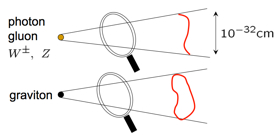String theory says that gauge particles such as photons, gluons, and W/Z bosons are open strings, but that the graviton is a closed string.
Kitazawa relates gravity and the gauge theory using superstring theory. In this theory, the gravitational interaction is an exchange of a string loop called graviton. When a loop is tossed, the trajectory of it would be cylindrical tube, so that the gravitational interaction takes the form of a cylinder in spacetime. There are two ways to look at the tube. One is a loop traveling through time, and the other is an open straight string going around in a circle. A closed string is a graviton and an open string is a gauge particle—such as photon, so the tube represents both gravity and gauge particles. “They are just two different ways to look at the same thing.”

Five superstring theories
String theory, when initially discovered, made mathematical sense only if there were 26 dimensions of spacetime. In this original form, string theory could only explain the force mediating particles, the bosons, and could not explain the other particle family, the fermions. As fermions are the particles from which matter is constructed, this was a problem. Physicists incorporated fermions into the theory by adding a property called supersymmetry. According to supersymmetry, every boson has a fermionic superpartner, and every fermion has a bosonic superpartner. The string theory that includes supersymmetry is known as superstring theory. Superstring is consistent in 10 dimensional spacetime.

With further study, physicists found that there are five distinct superstring theories which are consistent with our current knowledge of the universe. Individual strings could be open (a line with two ends) or closed (a loop). A closed loop could be ‘orientable’ or ‘unorientable’ depending on whether there was a defined direction along the length of the loop. In a racetrack in which cars can move in forward or backward direction, if the car can tell which direction it’s moving then the racetrack is orientable, while if the car cannot distinguish the direction it’s moving, the racetrack is said unorientable. An orientable closed string could also differentiate the left moving or right moving waves along the string. The superstring theory of open and closed strings is called Type I. The theories of closed orientable superstrings are called Type IIA and Type IIB where the former is left-right symmetric, while the latter is left-right asymmetric. The hybrid of left moving superstring and right moving bosonic string gives rise to two other superstring theories called heterotic strings. All five of these are supersymmetric, ten-dimensional, string theories.

The theory of particular interest here is the Type IIB superstring theory. The Type IIA strings turns out to produce a universe which is too symmetric. Our universe is filled with handedness. Neutrinos, for example, are left-handed. Type IIB superstring predicts an asymmetric universe, just like the one we live in.

Further developments in superstrings
Superstring physicists spent long years pondering, wondering which one of the five superstring theories would be the ultimate theory that they had been looking for. Then, in 1995, Edward Witten presented a unified string theory. He showed that all five superstring theories are different ways of looking at a bigger entity including an eleven dimensional theory called M-theory. The ‘M’ is said to stand for many things: ‘mother’, ‘mystery’, and ‘matrix’, among others.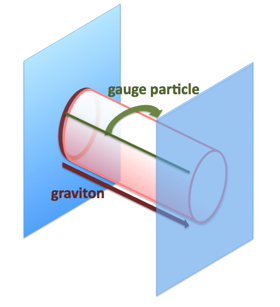A gravitational interaction between two D-branes. The graviton is the open red cylinder, while the D-branes are blue sheets. The cylindrical spacetime path of the graviton could be created either by a loop travelling parallel to the axis of the cylinder (tossed loop ,red), or by a longitudinal line moving tangentially around the circumference of the cylinder (green).At this same time, the matrix theory of superstrings emerged. Up to this point, string physicists had been using a perturbative approach, a mathematical method to approximate to the solution of a problem that could not be solved exactly. Unfortunately, this wasn’t working well, as the perturbed terms diverged to infinity. Therefore, physicists were looking for non-perturbative approach. This came in the form of the matrix model.

In the early 90s, Joseph Polchinski discovered that the superstring requires more extended objects called D-branes. This revolutionary idea was proved to be vastly successful, explaining microscopic structure of black-holes. According to Kitazawa, “It was conjectured D-branes be written by matrix models, which was a revolution in the field of string matrix model.”

IKKT matrix model
In 1996, four theorists at KEK, Ishibashi, Kawai, Kitazawa, and Tsuchiya developed a matrix model for the Type IIB superstring theory. This matrix model is called the IKKT model, and it in principle allows string theorists to solve the Type IIB superstring exactly to produce a description of spacetime.

“The matrix model has many degrees of freedom. In part, this means that the spacetime described by a matrix model is fuzzy,” explains Kitazawa. “That may sound a little odd at first, but it is a fundamental property of quantum mechanics. For example, the position and momentum of an electron cannot both be determined precisely at the same time because of the uncertainty principle.”

In quantum field theory, variables are defined at every point in spacetime to describe quantum fluctuations. This means that the theory is background dependent, that the details of the physics at a particular time and location are dependent on time and location. The physics of general relativity, on the other hand, is independent of background. A unified theory is expected to be independent of coordinate, so the dependence of field theory on coordinates has long been troubling to theorists. According to Kitazawa, the remarkable thing about “the matrix superstring model is that it is independent of coordinates.”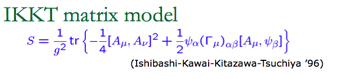The IKKT matrix model, discovered by KEK’s Ishibashi, Kawai, Kitazawa, and Tsuchiya, is equivalent to the Type IIB superstring theory. The discovery allows exact calculation of the theory.
Using the matrix model, physicists are trying to find a low-energy approximation to superstring theory, which they can then compare to the Standard Model. There are two ways to do this. First, one can use the traditional analytical approach, constructing the math and solving it by hand. Second, one can use the new numerical approach, and take advantage of the power of modern supercomputers.

Why four dimensions?
We live in four-dimensional spacetime. For physicists, the dimensionality is one of many questions that need to be answered. Why four, and not some other number? Neither general relativity nor quantum field theory can predict the dimensionality of spacetime. These theories require the number of dimensions be input as an empirical value. String theory, on the other hand, has ability to predict dimensionality. Although superstring theory predicts ten dimensions, the extra dimensions can be curled up in so small a volume that humans cannot perceive them, so that for all practical purposes, spacetime is four-dimensional. This is called compactification of dimensions.

When Kitazawa and Prof. Satoshi Iso of KEK apply the analytical approach to a matrix superstring model, it is by assuming solvable conditions of fields in spacetime. In that, they choose specific assumptions in order to make the calculation feasible. They have solved the matrix, for instance, assuming very low density of discrete spacetime points. Then they examine if the result would look like the spacetime we live in. “From the calculation of the simple case emerged a spacetime in which four-dimension is infinitely probable.” Because of the low spacetime point density they assumed, the space emerged as a very bumpy space, rather than a smooth one. They are looking for a new tool that allows them to further investigate the dimensionality of spacetime.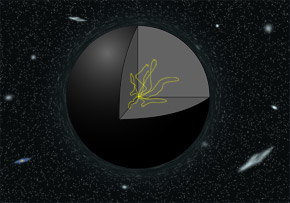Scientists thought it was impossible to compute the conditions inside a black hole until Prof. Jun Nishimura of KEK succeeded using a matrix superstring model.In addition to predicting the dimensionality of spacetime, superstring theory may also be able to predict other fundamental parameters such as the number of free constants and the number of generations of particles in the Standard Model. Iso says that, “superstring theory is deeply mathematical in nature. What distinguishes it from the empirical approach to physical problems is that we want to ‘explain’ numbers rather than putting them in a theory by hand.” By ‘explain,’ Iso means he hopes to derive values for fundamental physical constants from first principles. In short, he hopes to, “find the deeper mechanisms at work behind the current theory.”

Prof. Jun Nishimura recently announced some exciting news produced by computation of black hole thermodynamic properties using the matrix model. “I wanted to find something specific that could show that superstring theory is an accurate model of our reality,” says Nishimura. “Black holes, which are a manifestation of gravity, made this reality check possible.”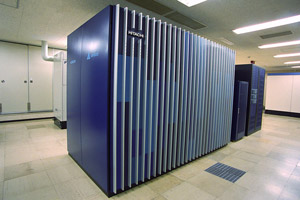Nishimura’s numerical calculation of the properties of a black hole required the use of this supercomputer at the KEK’s computing center.
Using general relativity and quantum field theory, Steven Hawking predicted that a black hole emits radiation called Hawking radiation. The detailed calculation of the dynamics inside a black hole was thought impossible a decade ago, because general relativity could not be applied to the center of black hole, where spacetime curvature diverges to infinity. Nishimura, who had been working on matrix theory computation for a long time, saw an opportunity. The result was dazzling: a perfect match with the prediction by Hawking.

“I would have been happy just to be able to show that the computation was possible,” says Nishimura. “Not only was I able to do that, but I was also able to reproduce the inner workings of black holes using a superstring matrix model.” The result was published in Physical Review Letters. His next challenge is to apply the similar calculation techniques to answer the question of four-dimensionality in the IKKT matrix model.

Not just the ultimate theory
Others have investigated additional ways to validate superstring theory. In 2005, an intriguing result came out of Brookhaven’s quark-gluon plasma experiment.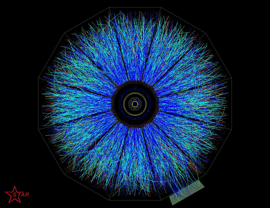A collision event in quark-gluon plasma. (Photo: Courtesy of Brookhaven National Laboratory)The viscosity of the quark-gluon plasma in the experiment was found to be surprisingly close to the value predicted by the superstring black hole calculation. According to the superstring theory, the quark-gluon plasma in four-dimensional spacetime is equivalent to a black hole in five-dimensional spacetime.

“This was the first time that string theory has ever been mentioned in an announcement of a major experiment,” says Dr. Makoto Natsuume who studies the quark-gluon plasma using black holes. “The gap between the predictions of superstring theory and the reality observed in the experiment is smaller than many of us had expected.”

The experiment also gave strong evidence that superstring theory can accurately explain the strong interaction. Iso says: “Superstring theory is not just the ultimate theory, but it also has the ability to make testable predictions for complex systems.”

The theory of particles, the universe, and everything
As the world center of study of Type IIB matrix theory, contributions of KEK’s superstring group to the worldwide superstring community are many. The individual interests of the group’s members are also very diverse. “Aside from the mathematical significance, superstring theory plays a role as the theory that unifies all theories of physics, and has many aspects,” says Iso. For Natsuume, superstring theory is an intellectual exercise, the theory is, “like a toy box, stuffed with many interesting theories, stimulating and fun.”

Dr. Shun’ya Mizoguchi, who studies reconstruction of the Standard Model from superstring theory, says he would likes to see where the beauty of the theory and the reality merge. “String theory is now different from when it started twenty years ago as the Theory of Everything. It has experienced several mathematical revolutions. Now that the Large Hadron Collider at CERN has started operation, it may be the right time to come back to where we started: particle physics.”copyright (c) 2010, HIGH ENERGY ACCELERATOR RESEARCH ORGANIZATION, KEK 1-1 Oho, Tsukuba, Ibaraki 305-0801 Japan | www-admin@kek.jp | Copyright | Send Question |